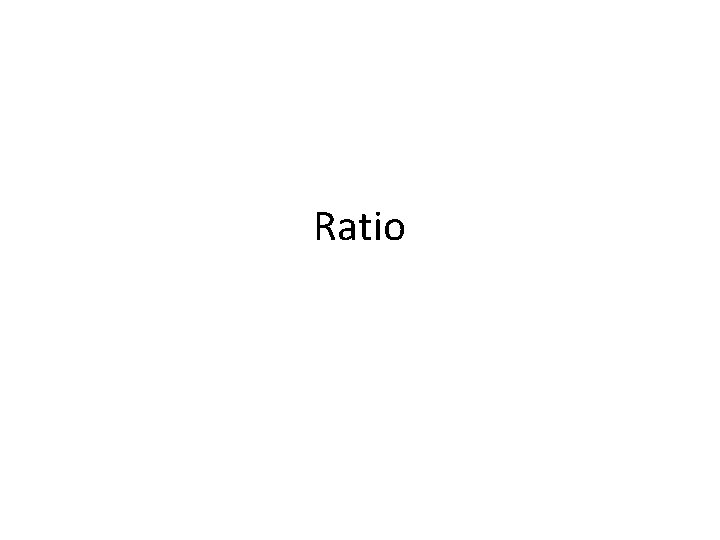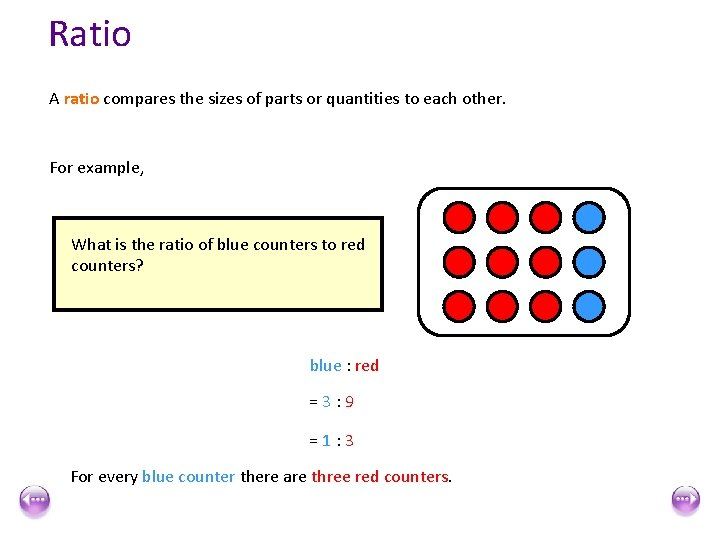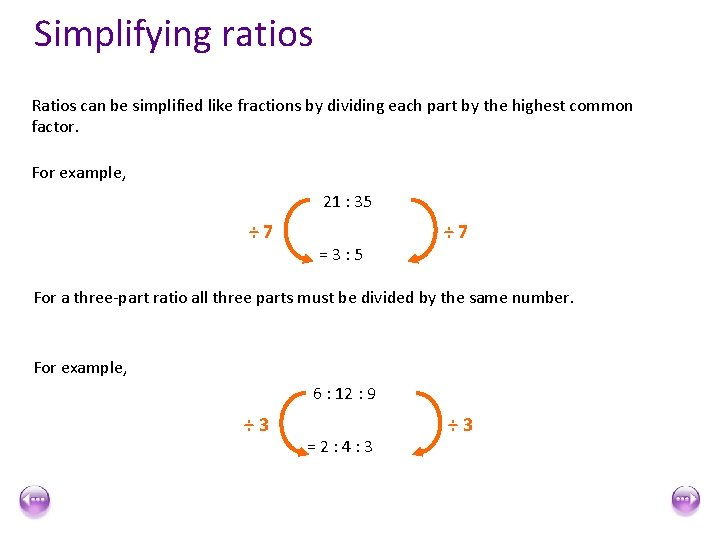# Ratio Ratio A ratio compares the sizes of

• Slides: 11RatioRatio A ratio compares the sizes of parts or quantities to each other. For example, What is the ratio of red counters to blue counters? red : blue =9: 3 =3: 1 For every three red counters there is one blue counter.Ratio A ratio compares the sizes of parts or quantities to each other. For example, The ratio of blue counters to red counters is not the same as the ratio of red What is the ratio of blue counters to red counters to blue counters? blue : red =3: 9 =1: 3 For every blue counter there are three red counters.Ratio What is the ratio of red counters to yellow counters to blue counters? red : yellow : blue = 12 : 4 : 8 = 3 : 1 : 2 For every three red counters there is one yellow counter and two blue counters.Simplifying ratios Ratios can be simplified like fractions by dividing each part by the highest common factor. For example, 21 : 35 ÷ 7 =3: 5 For a three-part ratio all three parts must be divided by the same number. For example, 6 : 12 : 9 ÷ 3 =2: 4: 3 ÷ 3Equivalent ratio spider diagramsSimplifying ratios with units When a ratio is expressed in different units, we must write the ratio in the same units before simplifying. Simplify the ratio 90 p : £ 3 First, write the ratio using the same units. 90 p : 300 p When the units are the same we don’t need to write them in the ratio. 90 : 300 ÷ 30 = 3 : 10 ÷ 30Simplifying ratios with units Simplify the ratio 0. 6 m : 30 cm : 450 mm First, write the ratio using the same units. 60 cm : 30 cm : 45 cm 60 : 30 : 45 ÷ 15 =4: 2: 3Simplifying ratios containing decimals When a ratio is expressed using fractions or decimals we can simplify it by writing it in whole-number form. Simplify the ratio 0. 8 : 2 We can write this ratio in whole-number form by multiplying both parts by 10. 0. 8 : 2 × 10 = 8 : 20 ÷ 4 =2: 5Simplifying ratios containing fractions Simplify the ratio : 4 2 3 We can write this ratio in whole-number form by multiplying both parts by 3. 2 3 : 4 × 3 = 2 : 12 ÷ 2 =1: 6Ratio 1. 2. 3. 4. 5. 6. 7. 8. ANSWERS There are 10 girls and 15 boys in a class, what is the ratio of girls to boys in its simplest 1. 2: 3 2. 7: 8 form? There are 14 cats and 16 dogs in an animal shelter, what is the ratio of cats to dogs in its 3. 2: 5 4. simplest form? a) There 22 caramels and 55 fudges in a bag of sweets, what is the ratio of caramels to fudges b) in its simplest form? c) Simplify these ratio to their simplest forms: d) e) a) 48: 60 5. b) 45: 75 a) c) 63: 108 b) d) 25: 40: 80 c) e) 24: 56: 96 6. f) 120: 180: 600 a) b) g) 320: 400: 440 c) Archie and Charlie share their Thomas the tank engine toys in the ratio 1: 4, how many do 7. they each get if they have: a) a. 10 toys b. 30 toys c. 45 toys b) Tom and Jerry share sweets in the ratio 2: 3, how many do they each get if they share: c) a. 20 sweets b. 30 sweets c. 55 sweets 8. a) Sue and Linda share some money in the ratio 3: 7, how many do they each get if they share: b) a. £ 30 b. £ 60 c. £ 90 c) Mike, Dave and Henry share some little bits of blue tack in the ratio 1: 2: 3, how many do they each get if they share: a. 60 pieces b. 72 pieces c. 300 pieces Home 4: 5 3: 5 7: 12 5: 8: 16 3: 7: 12 2 and 8 6 and 24 9 and 36 8 and 12 12 and 18 22 and 33 9 and 21 18 and 42 27 and 63 10 and 20 and 30 12 and 24 and 36 50 and 100 and 150